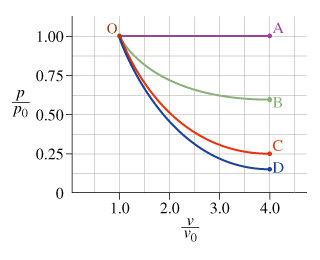# Problem: The following questions refer to the process represented by curve OB, in which an ideal gas proceeds from state O to state B.Part A. The pressure of the system in state B is __________ the pressure of the system in state O.A. greater thanB, less thanC. equal toPart B. The work done by the system is __________.A. greater than zeroB, less than zeroC. equal to zero

###### FREE Expert Solution

Part A

The curve OB represents an ideal gas equation.

The initial point of the curve OB is at the relative pressure 1 and the final point is at relative pressure 0.65.

91% (7 ratings)###### Problem DetailsThe following questions refer to the process represented by curve OB, in which an ideal gas proceeds from state O to state B.

Part A. The pressure of the system in state B is __________ the pressure of the system in state O.

A. greater than
B, less than
C. equal to

Part B. The work done by the system is __________.

A. greater than zero
B, less than zero
C. equal to zero